• 2019-10-16 16:50:20

## PSNR

### 1.峰值信噪比

一种评价图像的客观标准
最大值信号和背景噪音之间的关系
对于两个 m ∗ n m*n 的单色图像X和Y，其均方误差定义如下:
M S E = 1 m n ∑ i = 0 m − 1 ∑ j = 0 n − 1 ( X [ i , j ] − Y [ i , j ] ) 2 MSE = \dfrac{1}{mn} \sum_{i=0}^{m-1} \sum_{j=0}^{n-1}(X[i,j]-Y[i,j])^2

峰值信噪比定义如下，其中 M A X I MAX_I 表示图像像素点的最大值：
P S N R = 10 ∗ lg ⁡ ( M A X I 2 M S E ) PSNR = 10*\lg(\dfrac{MAX_I^2}{MSE})

### 2.彩色图像

针对彩色图像，通常用以下三种方法来计算。
1.分别计算 RGB 三个通道的 PSNR，然后取平均值。
2.计算 RGB 三通道的 MSE ，然后再除以 3 。
3.将图片转化为 YCbCr 格式，然后只计算 Y 分量也就是亮度分量的 PSNR。
其中，第二和第三种方法比较常见。本文实现采用了第二种方法。

### 3.结果分析

一般情况下：
PSNR高于40dB说明图像质量极好（即非常接近原始图像），
在30—40dB通常表示图像质量是好的（即失真可以察觉但可以接受），
在20—30dB说明图像质量差，
PSNR低于20dB图像不可接受。

PSNR代码实现

本文参考文章
 https://blog.csdn.net/leviopku/article/details/84586446
 https://www.cnblogs.com/seniusen/p/10012656.html

更多相关内容
• Python计算两图像的峰值信噪比PSNR 1.首先计算mse。 对于三通道的RGB图像 计算mse的数学表达式是： 转换成代码来写： 2.得到mse后计算PSNR。 转换为代码来写： ...

# 用Python计算两图像的峰值信噪比PSNR

图像信噪比是用于评估图像超分结果的指标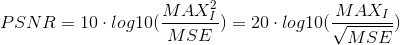def psnr(img1, img2):
mse = np.mean( (img1/255. - img2/255.) ** 2 )
if mse < 1.0e-10:
return 100
PIXEL_MAX = 1
return 20 * math.log10(PIXEL_MAX / math.sqrt(mse))

1.首先计算mse。

对于三通道的RGB图像 计算mse的数学表达式是：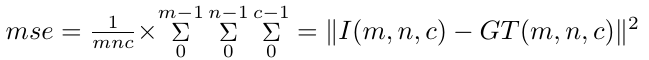转换成代码来写：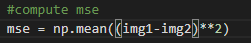2.得到mse后计算PSNR。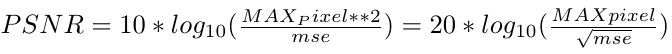转换为代码来写：

展开全文• 了解如何通过峰值信噪比来对图像质量进行客观评价，并计算峰值信噪比。 【实验环境】 (1) Windows XP 或 Vista 操作系统； (2) Python3.8、Pycharm 2021.2.2集成开发环境； (3) PNG 彩色无损压缩图像文件。 【原理...

# LSB图像隐写的python实现

### 【 实验目的】

了解信息隐藏中最常用的LSB算法特点，掌握LSB算法原理，设计并实现一种基于图像的LSB隐藏算法；了解如何通过峰值信噪比来对图像质量进行客观评价，并计算峰值信噪比。

### 【实验环境】

(1) Windows 7 以上操作系统；
(2) Python3.8、Pycharm 2021.2.2集成开发环境；
(3) PNG 彩色无损压缩图像文件。

### 【原理简介】

任何多媒体信息，在数字化时，都会产生物理随机噪，而人的感观系统对这些随机噪声不敏感。信息隐藏技术就是利用这个原理，通过使用秘密信息比特替换随机噪声，从而完成信息隐藏目标。
BMP 灰度图像的每个像素值为 8bit 二进制值，表示该点亮度。不同位平面对视觉影响不同，图像高位平面对图像感官质量起主要作用，去除图像最低几个位平面并不会造成画面质量的明显下降。利用这个原理可用秘密信息(或称水印信息)替代载体图像低位平面以实现信息嵌入。
本实验中算法选用最低位平面来嵌入秘密信息。最低位平面对图像的视觉效果影响最轻微，但很容易受噪声影响和攻击，解决办法可采用冗余嵌入的方式来增强稳健性。即在一个区域(多个像素)中嵌入相同的信息，提取时根据该区域中的所有像素判断。

### 【主要代码】

• 隐藏加密部分
from PIL import Image
def plus(st):
return st.zfill(8)
def get_key(strr):  # 实现对文字信息转成二进制
tmp = strr
with open(tmp,"r",encoding='utf-8') as f:
str = ''
for i in s:  # 这里i直接由文本转换成ASCII格式
if isinstance(i,int):
str = str+plus(bin(i).replace('0b',''))
else:
str = str+plus(bin(ord(i)).replace('0b',''))
return str
def mod(x,y): # 模除运算
return x%y
def encry(str1,str2,str3):
im = Image.open(str1)
width = im.size  # 获取图片的长,宽类似
print("length:"+str(width)+"\n")
height = im.size
print("width:"+str(height)+"\n")
count = 0
key = get_key(str2)
#     print(len(key))
keylen = len(key)
test=''
test2=''
for w in range(0,width):
if count == keylen:
break
for h in range(0,height):
pixel = im.getpixel((h,w))  # 获取指定长宽坐标像素的RGB值
#             print(pixel)
a = pixel  # R
b = pixel  # G
c = pixel  # B
if count == keylen:
break
test=test+str(mod(a,2))
a= a-mod(a,2)+int(key[count])
test2=test2+str(mod(a,2))
count += 1
if count == keylen:
im.putpixel((h,w),(a,b,c))  # 将指定坐标的像素值用（a，b，c）代替
break

test=test+str(mod(b,2))
b = b - mod(b,2) + int(key[count])
test2=test2+str(mod(b,2))
count += 1
if count == keylen:
im.putpixel((h,w),(a,b,c))
break

test=test+str(mod(c,2))
c = c - mod(c,2) + int(key[count])
test2=test2+str(mod(c,2))
count += 1
if count == keylen:
im.putpixel((h,w),(a,b,c))
break
if count % 3 == 0:
im.putpixel((h,w),(a,b,c))
im.save(str3)
print('嵌入前：'+test)
print('嵌入后：'+test2)


需要注意的是文本信息对应的编码格式要写对（错了好几次，难过上图是嵌入前的图片，下图是嵌入后的图片（能看出区别吗，不能。因为我放了两张一样的上去 LSB算法就是利用的视觉冗余原理，能看出来你就是下一个最强大脑啦）• 提取部分
def toasc(strr):
return int(strr,2)

def dest(le,str1,str2):  #le为所要提取的信息的长度，str1为加密载体图片的路径，str2为提取文件的保存路径
a = ''
b = ''
im = Image.open(str1)
length = le * 8
width = im.size
height = im.size
print("length:"+str(width)+"\n")
print("width:"+str(height)+"\n")
count = 0  # 计数，判断信息是否提取完毕

for h in range(0,height):
if count == length:
break
for w in range(0,width):
pixel = im.getpixel((w,h))
#             print('test:',int(str(pixel),2))
if count == length:
break
if count % 3 == 0:
count += 1  # 已经处理过了一位R/G/B上的信息
b = b + str(mod(int(pixel),2))  # 由于嵌入时，时按照RGB的顺序对每一位上的三个值进行嵌入，所以第一个要提取R上的信息
if count == length:  # 当长度与目标信息的一致时，退出
break
if count % 3 == 1:
count +=1
b = b + str(mod(int(pixel),2))
if count == length:
break
if count % 3 == 2:
count += 1
b = b + str(mod(pixel,2))
if count == length:
break
with open(str2,'w',encoding='utf-8') as f:
print('提取：'+b)
for i in range(0,len(b),8):
#             stra = ''
stra = toasc(b[i:i+8])
#             print('test: ',isinstance(stra,int))
#             print(stra.decode())
le = 30  # 由于未知长度，先设的大一些


结果是这样的：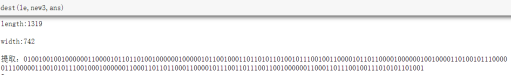经过解码得到隐藏信息如下

I am Admiral Hipper class cruiser

• 计算图像峰值信噪比
import cv2 as cv
import math
import numpy as np

def psnr1(img1,img2):
#compute mse
# mse = np.mean((img1-img2)**2)
mse = np.mean((img1/1.0-img2/1.0)**2)
#compute psnr
if mse < 1e-10:
return 100
psnr1 = 20*math.log10(255/math.sqrt(mse))
return psnr1

def psnr2(img1,img2):
mse = np.mean((img1/255.0-img2/255.0)**2)
if mse < 1e-10:
return 100
psnr2 = 20*math.log10(1/math.sqrt(mse))
return psnr2

imag1 = cv.imread('D://Machine Learning//data set//1234//3.jpg')# 图片路径不能有中文，否则会打不开，报错“ Nonetype……”
print (imag1.shape)
#print(imag2.shape)
# imag2 = imag2.reshape(352,352,3)
#print(imag2.shape)
res1 = psnr1(imag1,imag2)
print("res1:",res1)
res2 = psnr2(imag1,imag2)
print("res2:",res2)


关于import cv2报错的问题，主要在库的安装上
opencv-python的安装可以参考这篇文章

如果觉得有用的话，可以点一波关注呀~

展开全文pygame matlab 图像处理
• 峰值信噪比（PSNR） 峰值信噪比（Peak Signal to Noise Ratio ，PSNR）一种广泛使用的客观图像质量评价标准。取原始图像与失真图像间的均方差相对于 的对数值(其中 为每个采样值的比特数，8位图通常取255)。PNSR
• 1.首先计算mse。 对于三通道的RGB图像 计算mse的数学表达式是： 转换成代码来写： 2.得到mse后计算PSNR。 转换为代码来写： ...

1.首先计算mse。

对于三通道的RGB图像 计算mse的数学表达式是：转换成代码来写：2.得到mse后计算PSNR。转换为代码来写：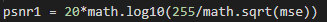或者将像素归一化：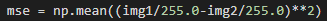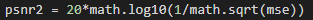理论上(数学意义上讲两个式子是相等的)，但实际结果是：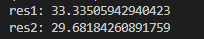两种计算方式的结果不同。原因是如果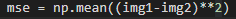中img1-img2的结果为负，那么得到的结果将是ASCII的补码，也就是，如果img1-img2是-2，那么输出将是256-2=254所以导致mse增大，如果img1某个点的像素比img2小，而两者差别又比较大，这个绝对值比较大的负值就会变成一个比较小的正值，mse的结果也会偏小，那么PSNR的值就会偏大。把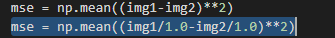上面的式子用下面的式子代替即可解决问题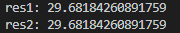完整的代码如下：

import cv2 as cv
import math
import numpy as np

def psnr1(img1,img2):
#compute mse
# mse = np.mean((img1-img2)**2)
mse = np.mean((img1/1.0-img2/1.0)**2)
#compute psnr
if mse < 1e-10:
return 100
psnr1 = 20*math.log10(255/math.sqrt(mse))
return psnr1

def psnr2(img1,img2):
mse = np.mean((img1/255.0-img2/255.0)**2)
if mse < 1e-10:
return 100
psnr2 = 20*math.log10(1/math.sqrt(mse))
return psnr2

print (imag1.shape)
#print(imag2.shape)
# imag2 = imag2.reshape(352,352,3)
#print(imag2.shape)
res1 = psnr1(imag1,imag2)
print("res1:",res1)
res2 = psnr2(imag1,imag2)
print("res2:",res2)


展开全文• PSNR的全称为“Peak Signal-to-Noise Ratio”，直译为中文就是峰值信噪比。 是一种衡量图像质量的指标。在很多领域都会需要这个指标，比如在超分辨率重建图像的时候，PSNR就是很重要的指标了。 WIKI解释 峰值...PSNR
• 峰值信噪比（英语：Peak signal-to-noise ratio，常缩写为PSNR）是一个表示信号最大可能功率和影响它的表示精度的破坏性噪声功率的比值的工程术语。由于许多信号都有非常宽的动态范围，峰值信噪比常用对数分贝单位来...代码
• Python-TF计算峰值信噪比PSNR以及相似性度量SSIM并批量输出保存为txt格式文件1.PSNR以及SSIM2.实现批量==图像对==PSNR、SSIM的计算。链接自己的其他可能用得上的博客1） 图片格式互换2）修改数据同名，方能测试 1....计算机视觉 深度学习
• PSNR的全称为“Peak Signal-to-Noise Ratio”，直译为中文就是峰值信噪比。 是一种衡量图像质量的指标。在很多领域都会需要这个指标，比如在超分辨率重建图像的时候，PSNR就是很重要的指标了。 WIKI解释 峰值...PSNR
• #计算峰值信噪比 def psnr(img1, img2): mse = np.mean( (img1/255. - img2/255.) ** 2 ) if mse < 1.0e-10: return 100 PIXEL_MAX = 1 return 20 * math.log10(PIXEL_MAX / math.sqrt(mse)) ori_img = cv2...opencv 图像识别
• PSNR（峰值信噪比）是单图像超分辨率重建中评价图像质量的一项重要指标，下面给出几种基于python语言的实现方法！ 公式推导： 给定一个大小为 m×n 的干净图像 I 和噪声图像 K ，均方误差 (MSE) 定义为： PSNR...
• 离散余弦变换，在（声音、图像）数据压缩中得到了广泛的使用。 求输入图像和经过离散余弦变换之后的图像的峰值信噪比。并求出离散余弦逆变换的比特率。均方误差 图像处理
• PSNR（Peak Signal-to-Noise Ratio 峰值信噪比） PSNR（Peak Signal-to-Noise Ratio），峰值信噪比，是一个表示信号最大可能功率和影响它的表示精度的破坏性噪声功率的比值的工程术语。由于许多信号都有非常宽的动态...计算机视觉 图像处理
• ## PSNR-峰值信噪比

千次阅读 2019-12-06 10:58:37
PSNR-峰值信噪比参考博客 PSNR的全称为“Peak Signal-to-Noise Ratio”，直译为中文就是峰值信噪比。是一种衡量图像质量的指标。在很多领域都会需要这个指标，比如在超分辨率重建图像的时候，PSNR就是很重要的指标了...
• PSNR的全称为“Peak Signal-to-Noise Ratio”，直译为中文就是峰值信噪比。 是一种衡量图像质量的指标。在很多领域都会需要这个指标，比如在超分辨率重建图像的时候，PSNR就是很重要的指标了。 解释 峰值信噪比 ...
• PSNR（峰值信噪比） 简介 Peak signal-to-noise ratio（简称PSNR）是一个工程术语，表示信号的最大可能功率与影响信号表示精度的干扰噪声功率之间的比值。由于许多信号都有非常宽的动态范围，峰值讯噪比常用对数分贝...psnr
• 主要介绍了python Opencv计算图像相似度过程解析,文中通过示例代码介绍的非常详细，对大家的学习或者工作具有一定的参考学习价值,需要的朋友可以参考下
• %求硬阈值去噪的峰值信噪比a1和信噪比a2 % RMSE=sqrt((sum((c-b).^2))./2); %软阈值结果评价 B=double(b); A=B-x1; MSE = sum(A(:).*A(:))/numel(B); %均方误差MSE，numel()函数返回矩阵元素个数 RMSE=sqrt(MSE); ...matlab
• 峰值信噪比，Peak signal-to-noise ratio（PSNR）是测量有损压缩编／解码器的重建质量的重要指标，在图像处理领域很常见，因为在图像压缩处理过程中，常常会引入噪声，这些噪声就会影响图像重建质量，对于图像重建，...深度学习 Tensorflow PSNR 代码
• SSIM和PSNR的python实现代码，可用于计算图片之间的差异，直接运行即可，有任何问题可以评论提出深度学习
• Python计算两张图的PSNR值     本文主要记录如何用python实现求解两张图的PSNR值。 目录Python计算两张图的PSNR值1.PSNR求解公式2.完整的PSNR求解代码3.运算结果展示4.参考文献 1.PSNR求解公式     （1）...算法...